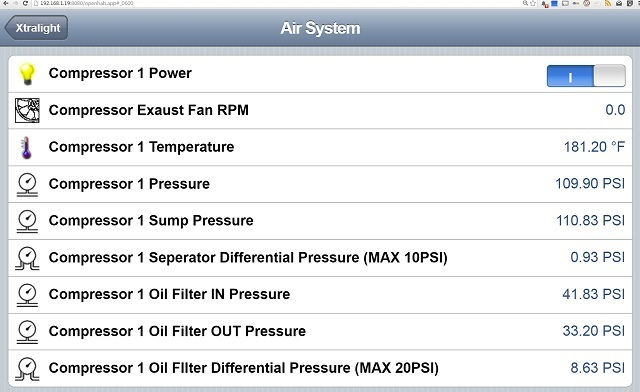# Conditional Calculation in rules

I have 2 pressure sensors that I read via modbus to determine the differential on a spereator while a compressor is running. One is on the output line that is after a check valve and the other is on the tank before the seperator. When the compressor is running the differential pressure is only about 1psi but when the compressor turns off the seperator tank pressure is vented quickly dropping. The other pressure sensor is on the line out still connected to the plant air lines and it depressurizes at a much slower rate the differential calculation reaches -90psi at times. that reading is irrelevant because the compressor is not running at the time but it messes up my charting when it dips that low.

What I would like to do is use the power swtch input ON/OFF to stop it from calculating and return 0 when the compressor is turned off.

Here are my current rules.

``````var Number span1 = 158.6 / 65535
var Number span2 = (-16.1)
var Number span3 = 158.4 / 65535
var Number span4 = (-16.1)

rule "modbus pressure scale"
when
Item COMP_1_MAIN_Pressure changed
then
var Number temp = COMP_1_MAIN_Pressure.state as DecimalType
temp = span1 * temp + span2
postUpdate(COMP_1_MAIN_Pressure_div, temp)

end

rule "modbus sump pressure scale"
when
Item COMP_1_SUMP_Pressure changed
then
var Number temp = COMP_1_SUMP_Pressure.state as DecimalType
temp = span3 * temp + span4
postUpdate(COMP_1_SUMP_Pressure_div, temp)

end

rule "modbus sump differential pressure"
when
Item COMP_1_SUMP_Pressure_div changed
or Item COMP_1_MAIN_Pressure_div changed
then
var Number temp1 = COMP_1_SUMP_Pressure_div.state as DecimalType
var Number temp2 = COMP_1_MAIN_Pressure_div.state as DecimalType
var temp = temp1 - temp2
postUpdate(COMP_1_SUMP_Diff, temp)

end
``````

Here are my items

``````Switch	COMP_1_MAIN_Power 		"Compressor 1 Power" 						(modbus,comp) 			{modbus="slave3:0"}
Number	COMP_1_MAIN_Pressure 		"Compressor 1 Pressure RAW" 					(modbus_raw) 			{modbus="slave4:0"}
Number	COMP_1_SUMP_Pressure 		"Compressor 1 Sump Pressure RAW" 				(modbus_raw) 			{modbus="slave4:1"}
Number	COMP_1_MAIN_Pressure_div	"Compressor 1 Pressure [%.2f PSI]"					<pressure>		(comp,CompGraphPres,Pressure,modbus,mod_agg)
Number	COMP_1_SUMP_Pressure_div	"Compressor 1 Sump Pressure [%.2f PSI]"					<pressure>		(comp,CompGraphPres,Pressure,modbus,mod_agg)
Number	COMP_1_SUMP_Diff		"Compressor 1 Seperator Differential Pressure (MAX 10PSI) [%.2f PSI]"	<diffpressure>		(comp,CompGraphPres,Pressure,modbus,mod_agg)
``````You can simply insert an if-clause to omit postUpdate, for example:

``````if(COMP_1_MAIN_Power.state==ON)
postUpdate(COMP_1_MAIN_Pressure_div, temp)
``````

so the item is only updated, if the compressor is on.

Yes I could but then the graph would stay the last updated value while it is off and not set to 0

I was thinking of something like when the value is 1/on on the power divide the value by one and when it is 0/off divide it by 0

You can’t divide by 0but surely you can set the pressure item to zero:
if(COMP_1_MAIN_Power.state==ON)
postUpdate(COMP_1_MAIN_Pressure_div, temp)
else
postUpdate(COMP_1_MAIN_Pressure_div, 0)

I ended up going a different route instead of using the switch I added an IF it is less than zero make it equal zero.

``````rule "modbus sump differential pressure"
when
Item COMP_1_SUMP_Pressure_div changed
or Item COMP_1_MAIN_Pressure_div changed
then
var Number temp1 = COMP_1_SUMP_Pressure_div.state as DecimalType
var Number temp2 = COMP_1_MAIN_Pressure_div.state as DecimalType
var temp = temp1 - temp2
if (temp<0)
temp=0
postUpdate(COMP_1_SUMP_Diff, temp)

end
``````